Chemguide: Support for CIE A level Chemistry ``` ``` Learning outcome 26: Reaction kinetics 26.1: Simple rate equations, orders of reaction and rate constants ``` ``` Learning outcome 26.1.3 This statement is about half-life in rate of reaction work. Before you go on, you should find and read the statement in your copy of the syllabus. ``` ``` Quite a lot of this is covered in detail in my chemistry calculations book. What follows is a brief summary. ``` ``` Concentration-time graphs for a first order reaction This was introduced in statement 26.1.2. First order reactions will have a curve if you plot concentration against time (but so will other orders as well - apart from zero order). A typical first order curve will look like this: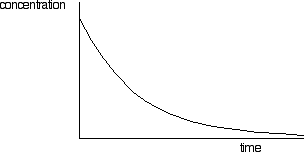But you can't tell it is first order just by looking at it. A second order curve isn't hugely different. It starts more steeply, but you need to investigate the curves more carefully to be sure. And this is where the concept of half-life comes in. The half-life of a reaction is the time it takes for the concentration of a substance to fall to half of its original value. For a first order reaction (but only for a first order reaction), the half-life is constant. It doesn't matter what concentration you start with, it will take exactly the same time for the concentration to reach half of that value. You can easily discover this from a graph of concentration against time. You simply have to measure how long it takes for the concentration to fall from its original value to half of that value; then measure it from a half to a quarter; then from a quarter to an eighth. Or you could measure it from the original 100% to 50%, and then compare that with the fall from 80% to 40%, and from 60% to 30% - or any other combination of concentrations, as long as you are measuring the time taken to halve the concentration. Looking at this on a graph:. . . you can see that the time taken to halve the concentration is always the same. If you did this for a reaction with some other order (second order, for example, or some fractional order), then the values won't be constant. This isn't a problem you need to worry about for CIE purposes. All you need to recognise is that only first order reactions have a constant half-life. ``` ``` The relationship between half-life and rate constant for a first order reaction Half-life is given the symbol t½. For first order reactions there is a simple relationship between this and the rate constant for the reaction, k.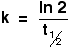This only applies to first order reactions. "ln 2" is the natural logarithm of 2. If you have no idea what that means, it doesn't matter in the least. Somewhere on your calculator is a button marked "ln". Most calculators probably want you to enter the number 2 and then press the ln button. It may be that yours does it the other way around. If you get it wrong you will just get an error message. You will find it gives an answer of 0.69314718. CIE round this off for you to 0.693, and actually expect you to remember the relationship as: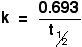Learn it! You can't do these simple sums without it. ``` ``` Example 1 If the half-life of a first order reaction is 300 seconds, calculate the rate constant for the reaction.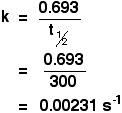Why are the units s-1? 0.693 has no units, and the units of half-life are seconds (s). The unit s was on the bottom of the fraction, and you need to write the units on the same line as the answer. To move the unit to the top, you have to change the sign, giving s-1. ``` ``` Example 2 If the rate constant of a first order reaction is 7.70 x 10-4 s-1, calculate the half-life of the reaction. First, you will have to rearrange the expression to let you calculate half-life.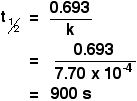``` ``` Provided you know the equation, these calculations are trivial. ``` ``` Go to the Section 26 Menu . . . To return to the list of learning outcomes in Section 26 Go to the CIE Main Menu . . . To return to the list of all the CIE sections Go to Chemguide Main Menu . . . This will take you to the main part of Chemguide. © Jim Clark 2020